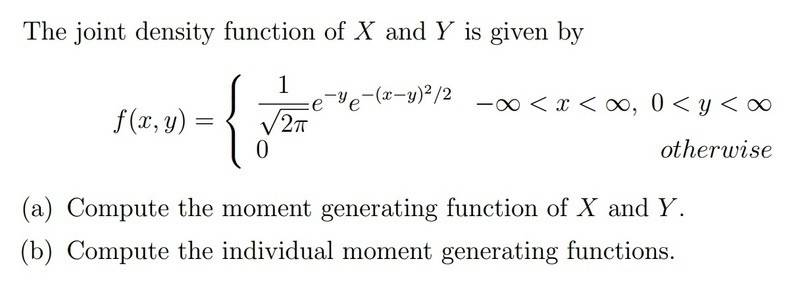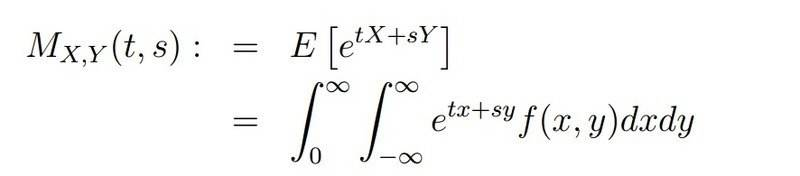# Finding the joint moment generating function given the joint PDF

## Homework Statement## The Attempt at a SolutionThe problem is the integral is non-elementary, so now what? Part (b) follows trivially from part (a). But is there some kind of shortcut I have to take, because no matter what substitution I do, the integral needs to be written in terms of the error function, which is out of the scope of this course. Any hints would be great. Thanks.

#### Attachments

maajdl
Gold Member
Try something.
Try integrating over x for example.
You will be surprised.

it seems something will just "vanish" but i can't find what, mainly because there is always the factor of e^x in there that gets in the way. I realize that it looks like a bivariate normal distribution though...

maajdl
Gold Member
Try integrating on x, as a start.
Since the integration spans [-Inf, +Inf], you will end up with a definite integral.
Alternatively, you might re-use known results on Laplace transforms.
See for example:

http://planetmath.org/laplacetransformofagaussianfunction

Proving what you find there is not difficult and could be interesting.

Other hint: 2bx + x² = (x+b)² - b²

Ray Vickson
Homework Helper
Dearly Missed

## Homework Statement## The Attempt at a SolutionThe problem is the integral is non-elementary, so now what? Part (b) follows trivially from part (a). But is there some kind of shortcut I have to take, because no matter what substitution I do, the integral needs to be written in terms of the error function, which is out of the scope of this course. Any hints would be great. Thanks.

Do the x-integration first; it has the form
$$G(t,y) = \int_{-\infty}^{\infty} e^{-y} e^{tx} e^{-(x-y)^2/2}/\sqrt{2 \pi} \, dx$$
Basically, this is a standard Gaussian integral, and it can be done in closed form because the integration limits are ##\pm \infty##. See. eg., http://en.wikipedia.org/wiki/Gaussian_integral .

to me, e^-(x-y)^2 looks like a gaussian integral. but with the extra e^(tx), it no longer looks like a "standard" gaussian integral. of course i can see that because the limits are +/- infinity, that the x integration is just gonna give root pi, or 1 or something stupid easy, but i'm not terribly familiar with WHAT the actual volume is under the 3d graph of a standard gaussian, let alone a gaussian with an extra e^(tx) in it...

wolfram gives

as the integral wrt x. i can't "see" that. but it looks like it follows from maajdl's suggestion....

Last edited by a moderator:
maajdl
Gold Member
to me, e^-(x-y)^2 looks like a gaussian integral. but with the extra e^(tx), it no longer looks like a "standard" gaussian integral. of course i can see that because the limits are +/- infinity, that the x integration is just gonna give root pi, or 1 or something stupid easy, but i'm not terribly familiar with WHAT the actual volume is under the 3d graph of a standard gaussian, let alone a gaussian with an extra e^(tx) in it...

First perform the first integral.
If you want to walk, you need a first step.
And you will see that the second will be much easier than the first step.

That's really a general rule: don't delay the first for the reason that you do not trust the second step.

maajdl
Gold Member
You didn't write here the step where you experience a difficulty.
I can a most guess what is your problem (if any).